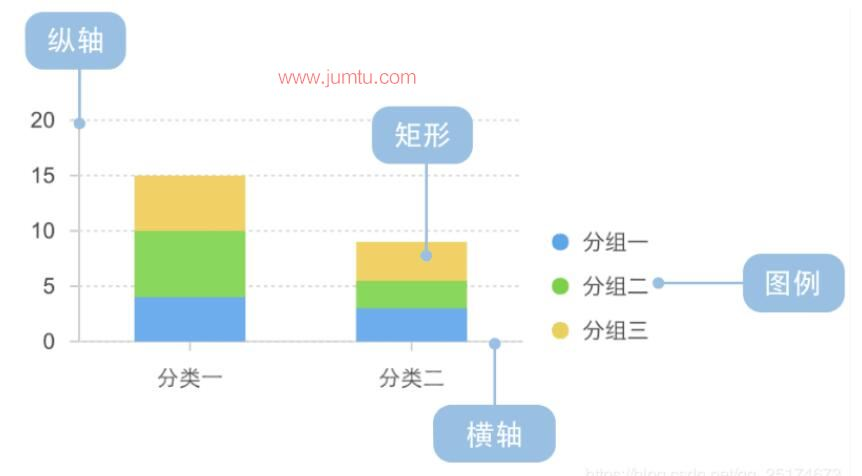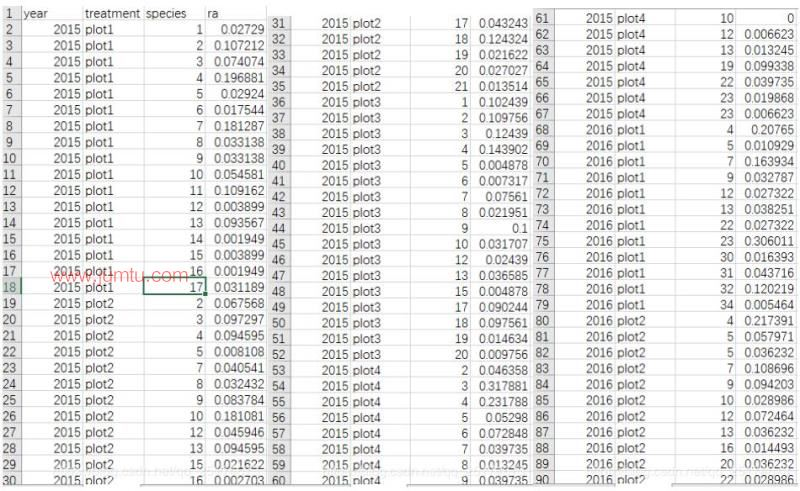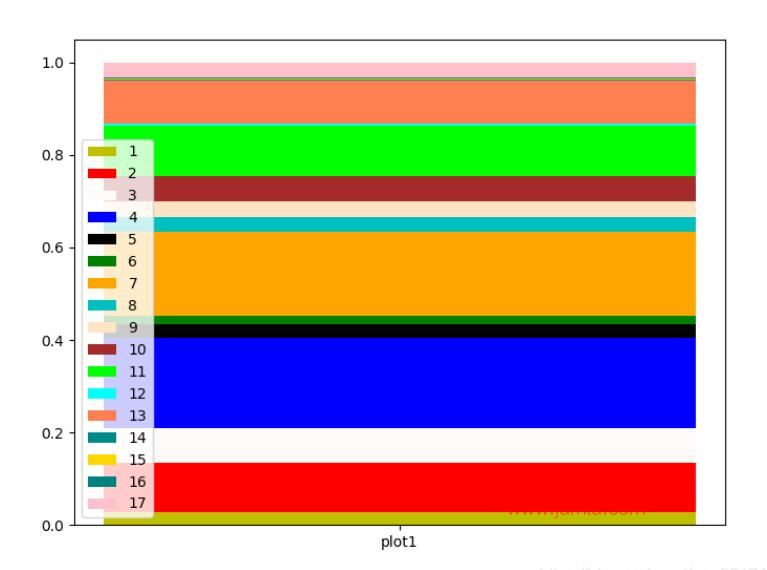• python堆积柱状图
千次阅读
2022-02-25 17:20:19
Axes.bar(self, x, height, width=0.8, bottom=None, *, align=’center’, data=None, **kwargs)


在绘制堆积图时，是在前一个的基础上累加的，即需要设置bottom参数。
举例：

import numpy as np
import matplotlib.pyplot as plt

labels = ['Month1', 'Month2', 'Month3', 'Month4']
mine = [21, 52, 33, 54]
others = [54, 23, 32, 41]
Mine_std = [2, 3, 4, 1]
Others_std = [3, 5, 2, 3]
width = 0.3

fig, ax = plt.subplots()

ax.bar(labels, mine, width,
yerr = Mine_std,
label ='Mine')

ax.bar(labels, others, width,
yerr = Others_std,
bottom = mine,
label ='Others')

ax.set_ylabel('Articles')
ax.legend()

ax.set_title('matplotlib.axes.Axes.bar Example')

plt.show()


其中第二个ax.bar（）参数设置bottom = mine即可。python
更多相关内容
• 堆积柱形图示例 涉及内容 数据堆积 #关键代码 y1 = (0,0,0,30.54,32.67,38.29,81.51) y2 = (87.83,85.07,92.14,57.29,52.4,53.85,14.55) d = [] for i in range(0, len(y2)): sum = y1[i] + y2[i] d.append...

# 涉及内容

• ## 数据堆积

#关键代码
y1 = (0,0,0,30.54,32.67,38.29,81.51)
y2 = (87.83,85.07,92.14,57.29,52.4,53.85,14.55)

d = []
for i in range(0, len(y2)):
sum = y1[i] + y2[i]
d.append(sum)
y3 = (12.17,14.93,7.86,12.17,14.93,7.86,3.94)
• ## 横向底部图例

#关键代码
plt.legend((p1, p2, p3), ('精确匹配', '粗匹配', '未匹配'),ncol=3,bbox_to_anchor=(0.5, -0.1), loc=8, borderaxespad=-3,frameon=False)

• ## 图片显示不完整

#关键代码（通过保存解决）
fig.savefig('pipeilv.jpg',bbox_inches='tight')
• ## 中文字体与英文字体

#关键代码（简便方法：折中找一个既像宋体，又像Times New Roman）
config = {
"font.family": 'STSong',
"font.size": 12,
"mathtext.fontset": 'stix',
"font.serif": ['STSong'],
}
rcParams.update(config)

# 重要参考

Python 画简单堆叠柱状图 （数据可视化） - 程序员大本营 (pianshen.com)

# 完整代码

import numpy as np
import matplotlib.pyplot as plt
import matplotlib
from matplotlib import rcParams
config = {
"font.family": 'STSong',
"font.size": 12,
"mathtext.fontset": 'stix',
"font.serif": ['STSong'],
}
rcParams.update(config)

fig=plt.figure(figsize=(8,4))#调整画布大小

N = 7
y1 = (15,17.22,19.63,30.24,34.49,40.84,70.5)
y2 = (17.14,14.26,15.71,25.08,22.85,23.61,23.5)

d = []
for i in range(0, len(y2)):
sum = y1[i] + y2[i]
d.append(sum)
y3 = (30.3,39.8,32.97,22.9,20,20.45,6)

d2 = []
for i in range(0, len(y2)):
sum = y1[i] + y2[i] +y3[i]
d2.append(sum)
y4=(28.36,19.82,24.49,12.98,12.94,10,0)

d3 = []
for i in range(0, len(y2)):
sum = y1[i] + y2[i] +y3[i] +y4[i]
d3.append(sum)
y5=(9.2,8.9,7.2,8.8,9.72,5.1,0)
# menStd = (2, 3, 4, 1, 2)
# womenStd = (3, 5, 2, 3, 3)
x = (1,2,3,4,5,6,7)  # the x locations for the groups
width = 0.35  # the width of the bars: can also be len(x) sequence

p1 = plt.barh(x, y1, width, color='#d62728')  # , yerr=menStd)
p2 = plt.barh(x, y2, width, left=y1)  # , yerr=womenStd)
p3 = plt.barh(x, y3, width, left=d)
p4=  plt.barh(x, y4, width, left=d2)
p5=  plt.barh(x, y5, width, left=d3,color='#7B7B7B')

plt.xlabel('位置精度区间分布(%)')
plt.ylabel('定位方式')
#plt.title('Scores by group and gender')
plt.yticks(x, ('百度 _G','腾讯 _G','高德 _G','百度  _S','腾讯  _S','高德  _S','所提方法'))
plt.xticks(np.arange(0, 110, 10))

plt.grid(which='major',axis='x',linestyle='dotted')

#plt.legend((p1, p2, p3,p4, p5), ('[0,50 m]', '(50 m, 200 m]', '(200 m, 500 m]','(500 m, 1000 m]','(1000 m, ∞)'))
plt.legend((p1, p2, p3,p4, p5), ('[0,50 m]', '(50 m, 200 m]', '(200 m, 500 m]','(500 m, 1000 m]','(1000 m, ∞)'),ncol=5,bbox_to_anchor=(0.5, -0.1), loc=8, borderaxespad=-3,frameon=False,handlelength=0.7)

plt.show()
fig.savefig('jingdu81.jpg',bbox_inches='tight')

展开全文python
• 本文目标在于利用 PythonPythonPython 快速画出符合自己要求的柱状图。 最近在处理某一组成绩数据的时候，涉及了柱状图的画法，因此此处进行一下记录。 加载库 import matplotlib.pyplot as plt import matplotlib....
• Python绘图库Matplotlib中并没有现成的函数可以一步绘制出堆积柱形图，所以这就需要我们通过灵活使用柱形图函数bar函数来实现。bar(x, height, width, bottom, *, align='center', **kwargs)bar函数中有四个参数是...

Python绘图库Matplotlib中并没有现成的函数可以一步绘制出堆积柱形图，所以这就需要我们通过灵活使用柱形图函数bar函数来实现。

bar(x, height, width, bottom, *, align='center', **kwargs)

bar函数中有四个参数是用来界定柱体位置及形状的，分别为x、height、width、bottom，它们分别代表柱体位置、柱体高度、柱体宽度、柱体底部位置。

这四个参数与柱体位置及形状关系示意图如下。通过示意图我们不难想到，我们可以通过分层绘制，以下一层高度作为上一层底部的方法来绘制堆积柱形图。

绘制时，除了最底层的不用设置底部参数bottom以外，其余每一层的bottom参数均为下面所有柱体的高度之和。

以07年至16年全国儿童、成人、老人人口总数为例，演示堆积柱形图绘制。11行之前的代码为变量的命名初始化。

9至11行：这里没有使用列表而是使用数组存储数据，是因为绘制堆积柱形图时需要对底层的柱体高度相加，如果采用列表存储数据，直接将两个列表相加得到是列表的拼接，而两个数组相加则是数组内元素一一对应相加，所以用数组存储数据更方便些。

16行：绘制儿童人口数柱体，由于是最底层，所以不需要设置bottom

17行：绘制第二层成年人人口数柱体，bottom参数设为儿童总人口数

18行：绘制第三层老年人人口数柱体，bottom参数设为儿童与成年人人口数之和

展开全文• 1.什么是堆叠柱状图与并排显示分类的分组柱状图不同，堆叠柱状图将每个柱子进行分割以显示相同类型下各个数据的大小情况。它可以形象的展示一个大分类包含的每个小分类的数据，以及各个小分类的占比，显示的是单个...

有个朋友要求帮忙绘制堆叠柱状图，查阅了一些文档之后也算是完成了，只是一个小demo，下面我就记录一下。

1.什么是堆叠柱状图

与并排显示分类的分组柱状图不同，堆叠柱状图将每个柱子进行分割以显示相同类型下各个数据的大小情况。它可以形象的展示一个大分类包含的每个小分类的数据，以及各个小分类的占比，显示的是单个项目与整体之间的关系。效果图如下：2.数据展示这里展示了部分数据，主要是treatment就是对应的上图分类一，分类二；species就是对应的分组；ra就是对应的各个分组的比例。

3.Python代码 from matplotlib import pyplot as plt

import pandas as pd

import xlrd

import numpy as np

data = xlrd.open_workbook('ccc.xlsx') #打开数据

table = data.sheet_by_index(0) #获取sheet1的数据

nrows = table.nrows #获取sheet1中的行

plot_list = ['plot1']

plot1_ra_list = [ for i in range(17)]#构建一个17X1的列表

i = 0

for row in range(1,nrows): #循环读取表内数据

if table.cell(row,0).value == 2015.0 and table.cell(row,1).value == 'plot1':

print(table.cell(row, 3).value) #第三列是各个元素所占的比例

print(i)

plot1_ra_list[i]=(float(table.cell(row, 3).value))

i+=1

#颜色列表

color = ['y','r','snow','b','k','g','orange','c','bisque','brown','lime','aqua','coral','darkcyan','gold','teal','pink',]

plt.figure(figsize=(8,6))

for i in range(17):

plt.bar(range(len(plot1_ra_list[i])), plot1_ra_list[i],bottom=np.sum(plot1_ra_list[:i],axis = 0),label=str(i+1),tick_label = plot_list,fc = color[i])

plt.legend()

plt.show()

4.效果展示

上述代码我只做了2015年的plot1，如果要把plot2增加上注意要对齐plot1和plot2的species数量，可以认为的添加，并补充对应的ra为0，这样子也不影响整个做图。下面我PO一张demo。以上这篇Python绘制堆叠柱状图的实例就是小编分享给大家的全部内容了，希望能给大家一个参考，也希望大家多多支持聚米学院。

展开全文• # 堆积图 import matplotlib.pyplot as plt import matplotlib as mpl mpl.rcParams["font.sans-serif"] = ["SimHei"] x = [1, 2, 3, 4, 5, 6 ,7,8,9,10,11,12,13,14] y = [0.4101,0.4535,0.4881,0.4604,0.4539,...
• python matplotlib 绘制百分比堆积柱状图python
• 堆积柱状图很常见，python matplotlib画出来的效果一点不比origin差，又比NCL方便太多。同时，画这幅图的时候还学到了一些matplotlib的小细节，所以把这个学习过程也记录了下来。 2. 代码解析 2.1 导入库 用到的库...python
•python
• 柱形图(又称柱状图、直方图、条形图)用于各组数据的数量比较，可以简单的分为水平柱形图、垂直柱形图堆积柱形图。本文详细介绍matpltlib中垂直、水平和堆积三种柱形图的绘制。我的公众号：“pythonic生物人“，...
•python
• 单击“可视化”窗格中的“堆积柱形图”图标， 在画布区域会出现堆积柱形图的模板， 由于没有填入数据， 因此堆积柱形图是灰色的。 2.在“字段”窗格中勾选“年龄”“性别”“学历”3 个字段， 在“可视化”窗格的...
• importmatplotlib.pyplot as pltimportmatplotlib as mplmpl.rcParams["font.sans-serif"] = ["SimHei"]mpl.rcParams["axes.unicode_minus"] =Falsex= [1, 2, 3, 4, 5]y= [6, 10, 4, 5, 1]y1= [2, 6, 3, 8, 5]plt.ba...
• 第三、堆积柱形图 第四、双向柱形图   柱形图：一种长方形的单位长度，根据数据大小回绘制的统计图，用来比较两个或以上的数据（时间或类别） 一、绘图函数 bar（left，right，width，color,bottom） barh...
• 折线+柱状图 别看这个图挺复杂的，其实只要准备好数据，设置好，这些响应式的浮窗、阴影全都是自动配置的，非常省心。 # name_list, bofang, danmu, zhuifan, pts_list都是list类型 # 它们的元素类型可以是'123'...python echarts
• 利用Python实现pandas结构数据中所有指定特征相关响应变量的百分比堆积柱状图，并标识数字。 首先一个较大的隐患是，代码中主要采用for循环，对运算效率存在影响，目前暂不调整。 #0# 意义： 对与日常办公需要绘制...python 人工智能
• 本文介绍了Python 堆叠柱状图绘制方法，分享给大家，具体如下：'''''''''''''''''''''''''''''''''''''''''''''''''''''''''''''''''''''''''''''''''''''''''''''''''''>>文件: 堆叠直方图.py>>作者: ...
• 快速绘制几种简单的柱状图。1垂直柱图（普通柱图）绘制普通柱图的python代码如下：（左右滑动可以查看全部代码）12345678910111213141516171819202122232425262728# -*- coding:utf-8 -*-# 申明编码格式为utf-8...
• 本文实例讲述了Python实现绘制双柱状图并显示数值功能。分享给大家供大家参考，具体如下：# -*- coding:utf-8 -*-#! python3import matplotlib.pyplot as pltimport mpl_toolkits.mplot3d#定义函数来显示柱状上的...
• 解决matplotlib中文显示问题. plt.rcParams[‘font.sans-serif’] = [‘SimHei’] plt.rcParams[‘axes.unicode_minus’] = False
• Python绘制堆叠柱状图 有个朋友要求帮忙绘制堆叠柱状图，查阅了一些文档之后也算是完成了，只是一个小demo，下面我就记录一下。 1.什么是堆叠柱状图 与并排显示分类的分组柱状图不同，堆叠柱状图将每个柱子进行...Python matplotlib
• plt.barh()表示绘制水平方向的柱状图，plt.bar()表示绘制竖直方向的柱状图。plt.scatter表示绘制散点图，plt.pie()表示绘制饼状图。 [太阳]选择题 以下哪一种方法可以画竖向柱状图？ A bar() B barh() C ...python java
• 通过Python中的matplotlib绘制百分比堆叠柱状图，并为每一个类别设置不同的填充图案。主要原因是有些论文打印出是黑白色的，不同类别之间区分不明显，所以做了这种方案。 存在一个问题：不知道如何根据填充图案设置...python matplotlib
• python柱状图容易，但是如何对不同柱子使用不同颜色呢？同时在柱子顶端显示精确数值？主要用的方法为：atplotlib.pyplot.bar(left, height, width=0.8, bottom=None, hold=None, data=None, **kwargs)参数说明：...
• Python绘图之柱形图绘制详解,定义,误差,代码,参数,斜纹Python绘图之柱形图绘制详解易采站长站，站长之家为您整理了Python绘图之柱形图绘制详解的相关内容。用python编程绘图，其实非常简单。中学生、大学生、研究生...
• 使用matplotlib创建百分比堆积柱状图的思路与堆积柱状图类似，只不过bottom参数累计的不是数值而是百分比，因此，需要事先计算每组柱子的数值总和，进而求百分比。未使用numpy版本适用于少量数据，数据结构需要手动...
• 我正在尝试使用python Matplotlib绘制堆积的条形，并且我要绘制正值和负值。我看过其他文章，谈论如何用正值和负值绘制堆积的条形，但是没有一个使用Matplotlib完成，所以我找不到解决方案。带有负JSON数据的......